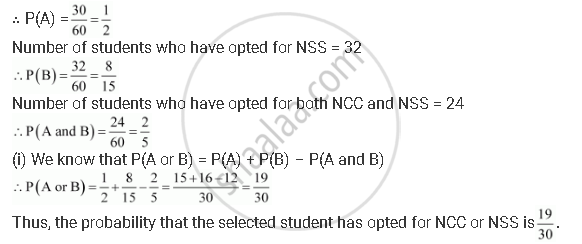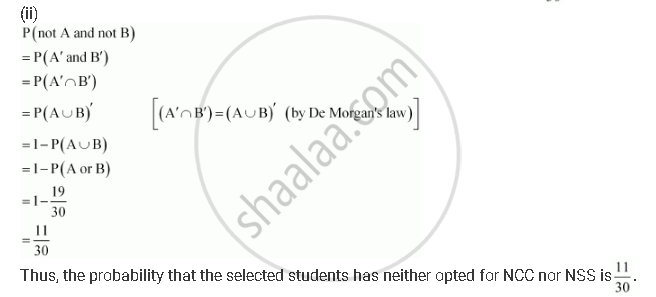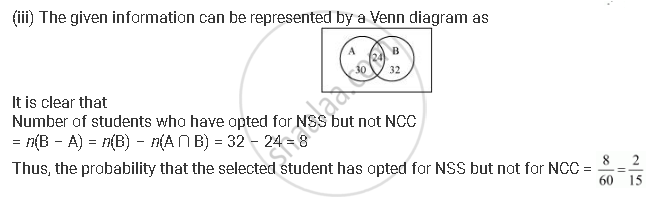# In a Class of 60 Students, 30 Opted for Ncc, 32 Opted for Nss and 24 Opted for Both Ncc and Nss. If One of These Students is Selected at Random, Find the Probability that - Mathematics

In a class of 60 students, 30 opted for NCC, 32 opted for NSS and 24 opted for both NCC and NSS. If one of these students is selected at random, find the probability that

(i) The student opted for NCC or NSS.

(ii) The student has opted neither NCC nor NSS.

(iii) The student has opted NSS but not NCC.

#### Solution

Let A be the event in which the selected student has opted for NCC and B be the event in which the selected student has opted for NSS.

Total number of students = 60

Number of students who have opted for NCC = 30Concept: Probability - Probability of 'Not', 'And' and 'Or' Events
Is there an error in this question or solution?

#### APPEARS IN

NCERT Class 11 Mathematics
Chapter 16 Probability
Q 21 | Page 406